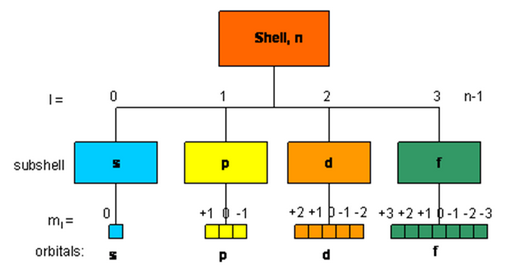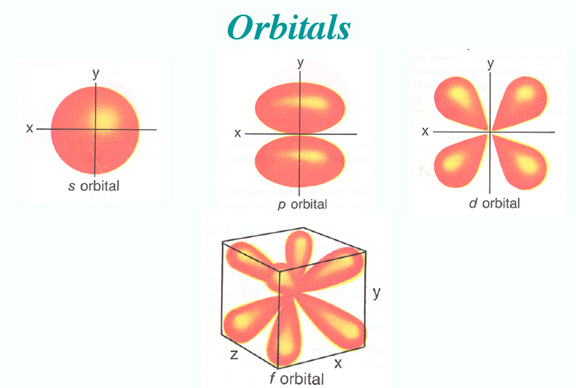Click to Chat

1800-1023-196

+91-120-4616500

CART 0

• 0

MY CART (5)

Use Coupon: CART20 and get 20% off on all online Study Material

ITEM
DETAILS
MRP
DISCOUNT
FINAL PRICE
Total Price: Rs.

There are no items in this cart.
Continue Shopping```Quantum Mechanical Model of Atom

Table of Content

Heisenberg’s Uncertainty Principle

Explanation of Heisenberg’s uncertainty principle

What is quantum mechanical model of the atom?

Nodal Points and Planes

Quantum Numbers?

Shapes and Size of Orbitals

Related Resources

Heisenberg’s Uncertainty PrincipleAll moving objects that we see around us e.g., a car, a ball thrown in the air etc., move along definite paths. Hence their position and velocity can be measured accurately at any instant of time. Is it possible for subatomic particle also?

As a consequence of dual nature of matter, Heisenberg, in 1927 gave a principle about the uncertainties in simultaneous measurement of position and momentum (mass x velocity) of small particles.

This Principle States:

“It is impossible to measure simultaneously the position and momentum of a small microscopic moving particle with absolute accuracy or certainty” i.e., if an attempt is made to measure any one of these two quantities with higher accuracy, the other becomes less accurate.

The product of the uncertainty in position (x) and the uncertainty in the momentum (p = m.v where m is the mass of the particle andv is the uncertainty in velocity) is equal to or greater than h/4π where h is the Planck’s constant.

Thus, the mathematical expression for the Heisenberg’s uncertainty principle is simply written asx.p > h/4π

Explanation of Heisenberg’s uncertainty principle

Suppose we attempt to measure both the position and momentum of an electron, to pinpoint the position of the electron we have to use light so that the photon of light strikes the electron and the reflected photon is seen in the microscope. As a result of the hitting, the position as well as the velocity of the electron are disturbed. The accuracy with which the position of the particle can be measured depends upon the wavelength of the light used. The uncertainty in position is ±λ. The shorter the wavelength, the greater is the accuracy. But shorter wavelength means higher frequency and hence higher energy. This high energy photon on striking the electron changes its speed as well as direction. But this is not true for macroscopic moving particle. Hence Heisenberg’s uncertainty principle is not applicable to macroscopic particles.

What is quantum mechanical model of the atom?

Atomic model which is based on the particle and wave nature of the electron is known as wave or quantum mechanical model of the atom. This was developed by Erwin Schrodinger in 1926. This model describes the electron as a three dimensional wave in the electronic field of positively charged nucleus. Schrodinger derived an equation which describes wave motion of an electron. The differential equation is

∂2ψ /∂x2 + ∂2ψ/∂y2 + ∂2ψ/∂z2 + 8π2m/h2 ( E - v ) ψ = 0

where x, y, z are certain coordinates of the electron, m = mass of the electron E = total energy of the electron. V = potential energy of the electron; h = Planck’s constant and ψ (psi) = wave function of the electron.

Significance of ψ: The wave function may be regarded as the amplitude function expressed in terms of coordinates x, y and z. The wave function may have positive or negative values depending upon the value of coordinates. The main aim of Schrodinger equation is to give solution for probability approach. When the equation is solved, it is observed that for some regions of space the value of ψ is negative. But the probability must be always positive and cannot be negative, it is thus, proper to use ψ2 in favour of ψ.

Significance of ψ2: ψ2 is a probability factor. It describes the probability of finding an electron within a small space. The space in which there is maximum probability of finding an electron is termed as orbital. The important point of the solution of the wave equation is that it provides a set of numbers called quantum numbers which describe energies of the electron in atoms, information about the shapes and orientations of the most probable distribution of electrons around nucleus.

Nodal Points and Planes

The point where there is zero probability of finding the electron is called nodal point. There are two types of nodes: Radial nodes and angular nodes. The former is concerned with distance from the nucleus while latter is concerned with direction.

No. of radial nodes = n – l – 1

No. of angular nodes = l
Total number of nodes = n – 1

Nodal planes are the planes of zero probability of finding the electron. The number of such planes is also equal to l.

Quantum Numbers?

An atom contains large number of shells and subshells (orbitals). These are distinguished from one another on the basis of their size, shape and orientation (direction) in space. The parameters are expressed in terms of different numbers called quantum numbers.

Refer to the following video for quantum numbers

Quantum numbers may be defined as a set of four numbers with the help of which we can get complete information about all the electrons in an atom. It tells us the address of the electron i.e., location, energy, the type of orbital occupied and orientation of that orbital.

Principal quantum number (n): It tells the main shell in which the electron resides, the approximate distance of the electron from the nucleus and energy of that particular electron. It also tells the maximum number of electrons that a shell can accommodate is 2n2, where n is the principal quantum number.

Shell

K

L

M

N

Principal quantum number (n)

1

2

3

4

Maximum number of electrons

2

8

18

32

Azimuthal or angular momentum quantum number (l): This represents the number of subshells present in the main shell. These subsidiary orbits within a shell will be denoted as 0, 1, 2, 3, 4,… or s, p, d, f… This tells the shape of the subshells. The orbital angular momentum of the electron is given as  √l(l +1) h/2π or √l(l+1) h  for a particular value of ‘n’ ( where h = h/2π). For a given value of n values of possible l vary from 0 to n – 1.

The magnetic quantum number (m): An electron due to its angular motion around the nucleus generates an electric field. This electric field is expected to produce a magnetic field. Under the influence of external magnetic field, the electrons of a subshell can orient themselves in certain preferred regions of space around the nucleus called orbitals. The magnetic quantum number determines the number of preferred orientations of the electron present in a subshell. The values allowed depends on the value of l, the angular momentum quantum number, m can assume all integral values between –l  to +l  including zero. Thus m can be –1, 0, +1 for l  = 1.Total values of m associated with a particular value of l is given by 2l+ 1.

The spin quantum number (s): Just like earth which not only revolves around the sun but also spins about its own axis, an electron in an atom not only revolves around the nucleus but also spins about its own axis. Since an electron can spin either in clockwise direction or in anticlockwise direction, therefore, for any particular value of magnetic quantum number, spin quantum number can have two values, i.e., +1/2 and –1/2 or these are represented by two arrows pointing in the opposite directions, i.e., ↑­ and ↓. When an electron goes to a vacant orbital, it can have a clockwise or anti clockwise spin i.e., +1/2 or –1/2. This quantum number helps to explain the magnetic properties of the substances.Quantum Number
Symbol
Values

Principal
n
1,2,...

Angular Momentum
l
0,1,2,...,n-1

Magnetic
m
-l to +l

Spin Magnetic
s
+1/2,-1/2

Shapes and Size of Orbitals

An orbital is the region of space around the nucleus within which the probability of finding an electron of given energy is maximum (90–95%). The shape of this region (electron cloud) gives the shape of the orbital. It is basically determined by the azimuthal quantum number l , while the orientation of orbital depends on the magnetic quantum number (m). Let us now see the shapes of orbitals in the various subshells.

s–orbital:These orbitals are spherical and symmetrical about the nucleus. The probability of finding the electron is maximum near the nucleus and keeps on decreasing as the distance from the nucleus increases. There is vacant space between two successive s–orbitals known as radial node. But there is no radial node for 1s orbital since it is starting from the nucleus.

p–orbital ( l=1):The size of the orbital depends upon the value of principal quantum number (n). Greater the value of n, larger is the size of the orbital. Therefore,
2s–orbital is larger than 1s orbital but both of them are non-directional and spherically symmetrical in shape.

The probability of finding the p–electron is maximum in two lobes on the opposite sides of the nucleus. This gives rise to a dumb–bell shape for the p–orbital. For p–orbital  l = 1. Hence, m = –1, 0, +1. Thus, p–orbital have three different orientations. These are designated as px, py & pz depending upon whether the density of electron is maximum along the x y and z axis respectively. As they are not spherically symmetrical, they have directional character. The two lobes of p–orbitals are separated by a nodal plane, where the probability of finding electron is zero.The three p-orbitals belonging to a particular energy shell have equal energies and are called degenerate orbitals.

d–orbital ( = 2):  For d–orbitals, l = 2. Hence m = –2,–1, 0, +1, +2. Thus there are 5d orbitals. They have relatively complex geometry. Out of the five orbitals, the three (dxy, dyz, dzx) project in between the axis and the other two dz2 and  dz2-y2  lie along the axis.Related Resources

Reference books of Physical Chemistry

IIT JEE Chemistry Syllabus

Electronic Configuration

To read more, Buy study materials of Structure of Atom comprising study notes, revision notes, video lectures, previous year solved questions etc. Also browse for more study materials on Chemistry here
```### Course Features

• 731 Video Lectures
• Revision Notes
• Previous Year Papers
• Mind Map
• Study Planner
• NCERT Solutions
• Discussion Forum
• Test paper with Video Solution